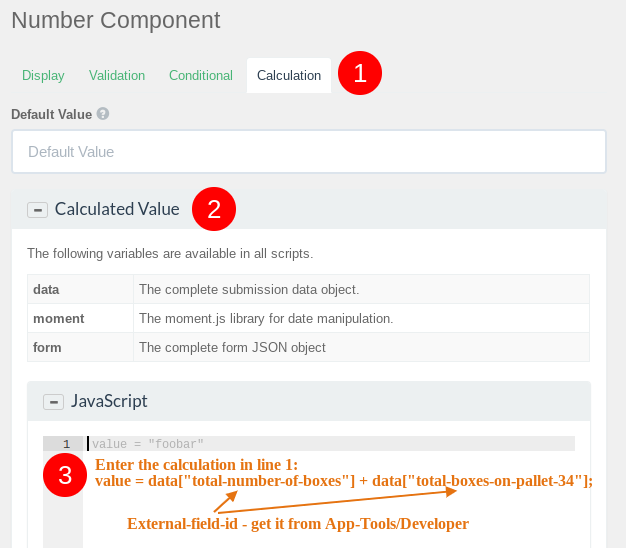1. Home
2. Knowledge Base
3. Dynamic Forms
4. Tips & Tricks
5. How to calculate the sum of multiple fields?

# How to calculate the sum of multiple fields?

Here is an example on how you can calculate the sum of multiple fields in a webform.

Example: The form has 2 editable number fields A and B and 3th number field C which is not editable. When the enters the number it should show automatically the sum (A+B) in field C.

In the field settings of field C click “Calculation” then open “Calculated Value” and enter this code (see screenshot):

`value = data["total-number-of-boxes"] + data["total-boxes-on-pallet-34"];`

The values in the square brackets are the external-field-IDs which you can get from the app (app menu/Developers).